```// Created by inigo quilez - iq/2013
// changed by stalendp@gmail.com
CGINCLUDE
#include "UnityCG.cginc"
#pragma target 3.0
struct vertOut {
float4 pos:SV_POSITION;
float4 srcPos;
};
vertOut vert(appdata_base v) {
vertOut o;
o.pos = mul (UNITY_MATRIX_MVP, v.vertex);
return o;
}
fixed4 frag(float4 sp:WPOS) : COLOR0 {
float2 p = (2.0*sp.xy - _ScreenParams.xy) / min(_ScreenParams.y, _ScreenParams.x);
p.y -= 0.25;
//background color
float3 bcol = float3(1.0,0.8,0.7-0.07*p.y) * (1.0 - 0.25*length(p));
// animate
float tt = fmod(_Time.y, 1.5)/1.5;
float ss = pow(tt,.2) * 0.5 + 0.5;
ss -= ss*0.2*sin(tt*6.2831*3.0)*exp(-tt*4.0);
p *= float2(0.5, 1.5) + ss * float2(0.5, -0.5);
// shape
float a = atan2(p.x,p.y)/3.141593;
float r = length(p);
float h = abs(a);
float d = (13.0*h - 22.0*h*h + 10.0*h*h*h)/(6.0-5.0*h);
// color
float s = 1.0-0.5*clamp(r/d,0.0,1.0);
s = 0.75 + 0.75*p.x;
s *= 1.0-0.25*r;
s = 0.5 + 0.6*s;
s *= 0.5+0.5*pow( 1.0-clamp(r/d, 0.0, 1.0 ), 0.1 );
float3 hcol = float3(1.0,0.5*r,0.3)*s;
float3 col = lerp( bcol, hcol, smoothstep( -0.01, 0.01, d-r) );
return float4(col,1.0);
}
ENDCG
Pass {
CGPROGRAM
#pragma vertex vert
#pragma fragment frag
#pragma fragmentoption ARB_precision_hint_fastest
ENDCG
}
}
FallBack Off
}
```

1. 心形画法的原理

float a = atan2(p.y,p.x)/3.1415;

atan2(p.y,p.x)求的是向量(x,y)所对应的角度。求得的是弧度制的值，除以pi后得到的范围是［0，1］；

``` float a = atan2(p.y,p.x)/3.141593;
float r = length(p);
float3 col = lerp( bcol, hcol, smoothstep( -0.01, 0.01, a-r) ); // 当p1时，smoothstep返回1；p2时，smoothstep返回0；
```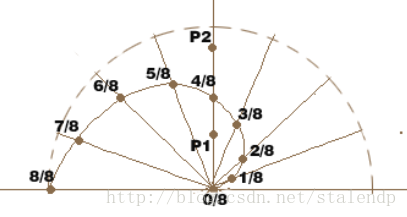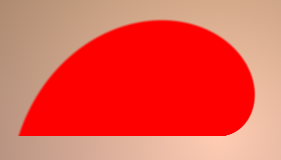```float a = atan2(p.x,p.y)/3.141593;
float r = length(p);
float h = abs(a);
float3 col = lerp( bcol, hcol, smoothstep( -0.01, 0.01, h-r) );```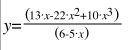，对应的曲线如下：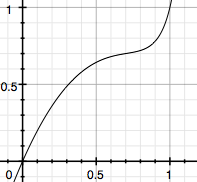2. 心形跳动的算法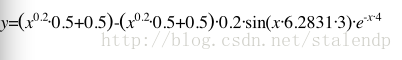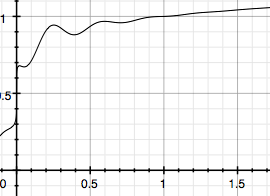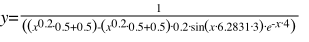```// animate
float tt = fmod(_Time.y, 1.5)/1.5; // 周期为1.5秒
float ss = pow(tt,.2) * 0.5 + 0.5;
ss -= ss*0.2*sin(tt*6.2831*3.0)*exp(-tt*4.0);
p *= float2(0.5, 1.5) + ss * float2(0.5, -0.5); // 不同的轴影响不同，使得心在跳动时，纵向变矮，横向变宽```

3. 颜色（待续）